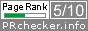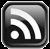# LEARN MS-ACCESS TIPS AND TRICKS

Learn Microsoft Access Advanced Programming Techniques, Tips and Tricks.

### Introduction.

How to find the week-end date or first-day date of a week using the Current Date as input.  Or find the first/last-day date of any week by giving a date value as input.

This kind of calculation may become necessary to find the week-end date and use it as criteria in queries, filter data for viewing, or printing of Reports for sharing of information.

Wherever you need it you can use the following simple expression to find the week-end date of the current week:

### The Simple Expressions

```LastDayOfWeek = Date()-WeekDay(date())+7
```

If the current date is 14th June 2017 (or any date between 11 and 17 June 2017) then the value returned in variable LastDayOfWeek = 17th June 2017.

#### Example-1

To find the first-day date of the current week uses the following method:

```FirstDayOfWeek = date()-WeekDay(date())+1
```

Assuming the current date is 14th June 2017 (or any date between 11 and 17 June 2017) the first-day date of the week returned in variable FirstDayofWeek = 11th June 2017.

#### Example-2

By giving a specific date as input to the expression to find the last-day date of the week:

```dtValue = #06/27/2017#
LastDayOfWeek = dtValue - WeekDay(dtValue)+7
Result: 1st July 2017```

#### Example-3

If you would like to do it differently then try the following expression:

```x = #06/27/2017#
'To find the last-day date of the Week:
LastDayofWeek = DateSerial(Year(Date()),1,1)+DatePart("ww",x)*7-1
Result: 1st July, 2017
'To find the first-day date of the Week.
FirstDayofWeek = DateSerial(Year(Date()),1,1)+DatePart("ww",x)*7-7
Result: 25th June, 2017```

### WeekLastDay Function

Define it as a Function in a VBA Module and call it wherever you want, with a date value as the parameter. Sample Function code is given below:

```Public Function WeekLastDay(ByVal dtVal As Date) As Date
'Date value as input
WeekLastDay = dtVal - WeekDay(dtVal) + 7
End Function```

### WeekFirstDay Function

```Public Function WeekFirstDay(ByVal dtVal As Date) As Date
'Date value as input
WeekFirstDay = dtVal - WeekDay(dtVal) + 1
End Function
```
Share:

Comments subject to moderation before publishing.

## TranslateSubscribe in a readerYour email address:

Delivered by FeedBurner